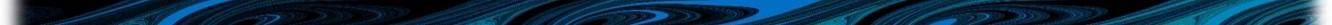# Appendix M Processes: phyto other

Photosynthetically available radiation is provided to the WQ Module from TUFLOW for each computational cell at each water quality timestep. It is this two- or three-dimensional field that the WQ Module uses to compute phytoplanktonic responses to light. The WQ Module allows, however, for modification of this TUFLOW field to incorporate the effect of light attenuation due to the presence of phytoplankton. This additional light extinction is computed dynamically at each water quality timestep, based on instantaneous phytoplanktonic concentrations in individual computational cells, as per Equation (M.1).

$\begin{equation} Ke_{\langle computed \rangle}^{phy} = Ke^{phy} \times \left[PHY\right] \tag{M.1} \end{equation}$

For each phytoplankton group, $$Ke^{phy}$$ is the user specified (or default) self shading coefficient and $$\left[PHY\right]$$ is the instantaneous phytoplankton concentration in each cell. For each cell and at each timestep, this quantity $$Ke_{\langle computed \rangle}^{phy}$$ is computed for each phytoplankton group. Results are then summed within each cell and returned to TUFLOW as a two- or three-dimensional field which TUFLOW then includes these in subsequent light distribution calculations.

## M.2 Cell density

The principle of the WQ Module density calculations is that cell density is set to increase during daylight primary production (when carbohydrates are created via photosynthesis) and decrease during darkness respiration (when carbohydrates are consumed). Equations (M.2) and (M.3) are used to describe these processes, respectively.

$\begin{equation} \rho_{PHY\langle computed \rangle} = \rho_{PHY} + \frac{0.124 \times \left(1.0 - e^{\frac{-PAR}{I_K}}\right) - 0.0230}{60} \tag{M.2} \end{equation}$

$\begin{equation} \rho_{PHY\langle computed \rangle} = \rho_{PHY} - \frac{0.0230}{60} \tag{M.3} \end{equation}$

$$\rho_{PHY\langle computed \rangle}$$ and $$\rho_{PHY}$$ are the computed and initial (previous timestep) cell densities, respectively and $$PAR$$ and $$I_K$$ are the ambient cell photosynthetically active radiation and half saturation light intensity, respectively. $$I_K$$ is specified via the light limitation function, or if not, then via the specification of the stokes settling model, or as the default. Computed cell densities are checked against specified (or default) global minima and maxima and reset to these limits if exceeded. Cell density is only used within the WQ Module to compute stokes settling, and need not be included as a computed variable if other settling models are deployed.